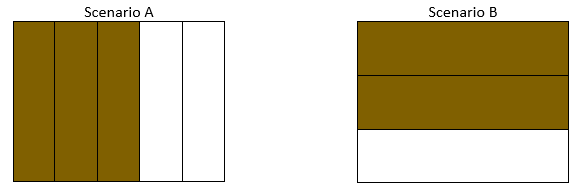# Fraction Equivalence and Ordering

## Objective

Compare two fractions with unrelated numerators and denominators by finding common units or number of units.

## Common Core Standards

### Core Standards

?

• 4.NF.A.2 — Compare two fractions with different numerators and different denominators, e.g., by creating common denominators or numerators, or by comparing to a benchmark fraction such as 1/2. Recognize that comparisons are valid only when the two fractions refer to the same whole. Record the results of comparisons with symbols >, =, or <, and justify the conclusions, e.g., by using a visual fraction model.

?

• 3.NF.A.3.D

## Criteria for Success

?

1. Compare two fractions where both fractions need to be replaced with an equivalent one to be able to compare common units (denominators) or common number of units (numerator) (MP.3).
2. Understand that there are a variety of common units or number of units that can be compared, including the possibility to find the largest possible unit to compare (i.e., the lowest common denominator).
3. Use the correct symbol (<, >, =) to record a comparison.
4. Understand that comparisons are valid only when the two fractions refer to the same whole.

## Tips for Teachers

?

As the Fractions Progressions states, “it is not necessary to find a least common denominator to calculate sums of fractions, and in fact the effort of finding a least common denominator is a distraction from understanding algorithms for adding fractions” (NF Progressions, p. 11). One can extend this rationale to finding a least common denominator to compare fractions and thus should not be overemphasized. However, because finding a least common denominator connects the supporting work of gaining familiarity with factors and multiples (4.OA.4) with fraction equivalence and comparison (4.NF.A), the idea is discussed briefly, particularly in Anchor Task #3. However, because finding a least common denominator connects the supporting work of gaining familiarity with factors and multiples (4.OA.4) with fraction equivalence and comparison (4.NF.A), the idea is discussed briefly, particularly in Anchor Task #3.

#### Fishtank Plus

• Problem Set
• Student Handout Editor
• Vocabulary Package

?

### Problem 1

Would you rather have the leftover brownies in Scenario A or Scenario B? The pans in which the brownies were cooked are the same size.### Problem 2

Compare the following fractions.

a.     ${{2\over3}}$ and ${{5\over8}}$

b.     ${{7\over4}}$ and ${{9\over5}}$

### Problem 3

Compare the following fractions.

a.     ${{7\over12}}$ and ${{5\over8}}$

b.     ${{13\over10}}$ and ${{5\over4}}$

## Problem Set & Homework

#### Discussion of Problem Set

• In #1, were some comparisons easier than others? Why?
• In #3, did you need to use multiplication for every part? Why or why not? When is multiplication not needed, even with different denominators?
• In #3(b), did everyone use forty-eighths? Did anyone use twenty-fourths?
• In #5, how did you compare the fractions? Why?
• Do we always need to multiply the denominators to make like units?

?

Compare the following fractions by writing <, >, or = in the blank.

1.     ${{3\over4}}$     _____________     ${{4\over5}}$

2.     ${{4\over6}}$     _____________     ${{3\over5}}$

#### References

EngageNY Mathematics Grade 4 Mathematics > Module 5 > Topic C > Lesson 15Exit Ticket, Question #1

Grade 4 Mathematics > Module 5 > Topic C > Lesson 15 of the New York State Common Core Mathematics Curriculum from EngageNY and Great Minds. © 2015 Great Minds. Licensed by EngageNY of the New York State Education Department under the CC BY-NC-SA 3.0 US license. Accessed Dec. 2, 2016, 5:15 p.m..

Modified by The Match Foundation, Inc.

?